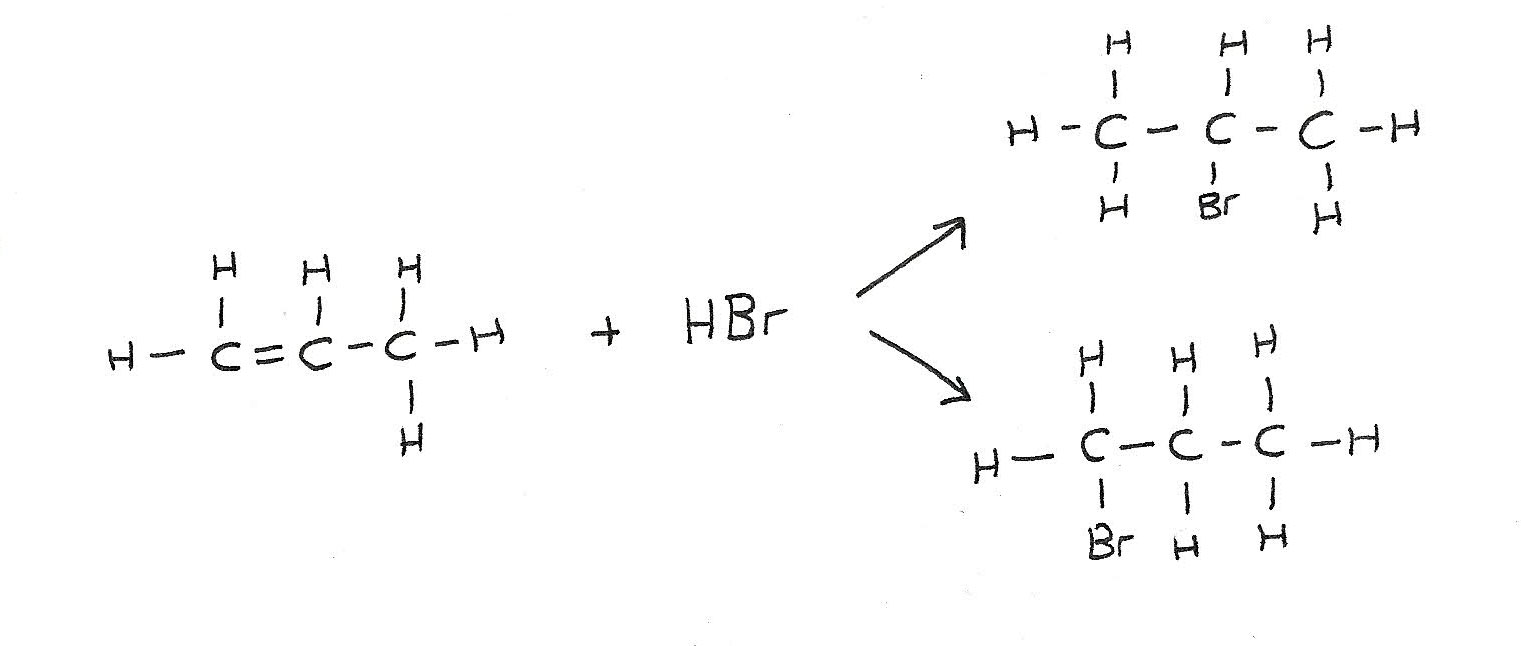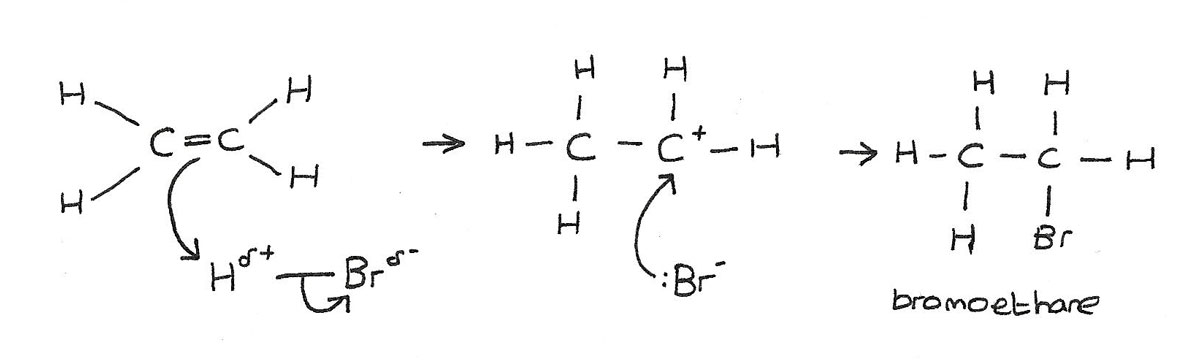# Alkenes

Alkenes are unsaturated hydrocarbons with at least one $C=C$ double bond. Alkenes:

• With one double bond have a general formula $C_{n}H_{2n}$.
• Are more reactive than alkanes and typically take part in addition reactions.
• Can form E/Z isomers, including cis/trans isomers.

### Ethene

$CH_{2}=CH_{2}$ is one of the most important alkenes. It is obtained from the catalytic cracking of crude oil fractions. The $C=C$ double bond is the functional group in the alkene that is responsible for its reactions.
$$Cl_{2} \rightarrow Cl\cdot + Cl\cdot$$

## Nature of the double bond

The $C=C$ bond is made up of two parts: a sigma ($\sigma$) bond and a pi ($\pi$) bond.

• A $\sigma$ bond is formed directly between the carbon atoms by the overlap of orbitals. Each carbon atom contributes one electron to the electron pair in the bond.
• The $\pi$ bond is formed above and below the plane of the carbon atoms by the sideways overlap of p-orbitals. Each carbon atom contributes one electron from a p-orbital to the electron pair in the $\pi$ bond.

In alkenes, each carbon involved in the double bond:

• Uses three of its electrons in the formation of $\sigma$ bonds.
• Uses one of its electrons in the formation of the $\pi$ bond.### Shape of an alkene molecule

• Three regions of high electron density surround each carbon atom in the $C=C$ double bond.
• Pairs of electrons repel each other as far apart as possible.
• The electron pairs repel each other to give the molecule a trigonal planar shape, with bond angles of around 120°.

A mixture of hydrogen gas $H_{2}$ and a gaseous alkene is passed over a catalyst of nickel at a temperature of 150°. This is sometimes known as a hydrogenation reaction.
$$CH_{2}{=}CH_{2} + H_{2} \rightarrow CH_{3}CH_{3}$$

Alkenes react rapidly with halogens such as chlorine, bromine and iodine at room temperature. This reaction sometimes known as a halogenation reaction, forms a di-substituted halogenoalkane.
$$CH_{2}{=}CH_{2} + Cl_{2} \rightarrow CH_{2}ClCH_{2}Cl$$

A hydrogen halide can be added across the double bond of an alkene to produce a halogenoalkane. Halogen halides include $HCl$, $HBr$ and $HI$. At room temperature, hydrogen halides are gases and can be bubbled through liquid alkenes.
$$CH_{2}{=}CH_{2} + HBr \rightarrow CH_{3}CH_{2}Br$$

Unsymmetrical alkenes such as propene can form a mixture of organic products. This is because the atoms can be added at either side of the double bond.The addition of steam to alkenes is one method of producing alcohols. This reaction is widely used in industry.

• Steam and a gaseous alkenes are heated to a high temperature and pressure in the presence of a phosphoric acid catalyst.
• This process is sometimes known as the hydration of alkenes.
$$CH_{2}{=}CH_{2} + H_{2}O \rightarrow CH_{3}CH_{2}OH$$

• The $C=C$ double bond in alkenes represent an region of high electron density. This density is due to the $\pi$ electrons in the double bond.
• Electrophiles are attracted to these $\pi$ bonds. This reaction of alkenes is an electrophilic addition reaction.

Hydrogen bromide, $HBr$ is a polar molecule. This is because bromine is more electronegative than hydrogen. This causes the dipole $H^{\delta+}{-}Br^{\delta-}$

• The electron pair in the $\pi$ bond is attracted to the slightly positive hydrogen atom, causing the double bond to break.
• A new bond is formed between one of the carbon atoms and the hydrogen atom.
• The $H{-}Br$ bond breaks by heterolytic fission, with the electron pair going to the bromine.
• A bromine ion $Br^-$, and a carbocation $C^+$ are formed.
• The positively charged carbocation is unstable and quickly reacts with the negatively charged bromine ion to form the organic product.The product of the reaction is a halogenalkane, in this case bromoethane. In the mechanism above, $HBr$ is the electrophile.

Bromine reacts with alkenes at room temperature, producing a di-substituted halogenoalkane.

• Bromine is a non-polar molecule. Upon approaching an alkene however, the electrons in the $\pi$ bond repel the electrons in the $Br{-}Br$ bond.
• This induces a dipole in the $Br_{2}$ molecule which is now slightly polar: $Br^{\delta+}{-}Br^{\delta-}$.
• The electron pair in the $\pi$ bond is attracted to the slightly positive bromine atom causing the $C{=}C$ double bond to break.
• A new bond forms between one of the carbon atoms and the bromine atom. The $Br{-}Br$ bond breaks by heterolytic fission, with the electron pair going to one bromine atom.
• A bromine ion $Br^-$ and carbocation $C^+$ are formed.
• The carbocation attracts the bromine ion forming a new $C{-}Br$ bond.

### Test for unsaturation

When $Br_{2(aq)}$ is added to an alkene the colour changes from orange to colourless. This colour change indicates that it has reacted with a alkene.

## Manufacture of margarine

• Vegetable oils are liquids containing long hydrocarbon chains, often with many double bonds and are polyunsaturated.
• The oil must be hardened to allow for easy spreading. This is done through the process of hydrogenation.
• This adds hydrogen atoms across the double bonds which partially solidifies and hardens the oils.
• Partial hydrogenation of unsaturated fats can transform some cis double bonds into trans double bonds. These trans fats are now thought to be bad for people's health.